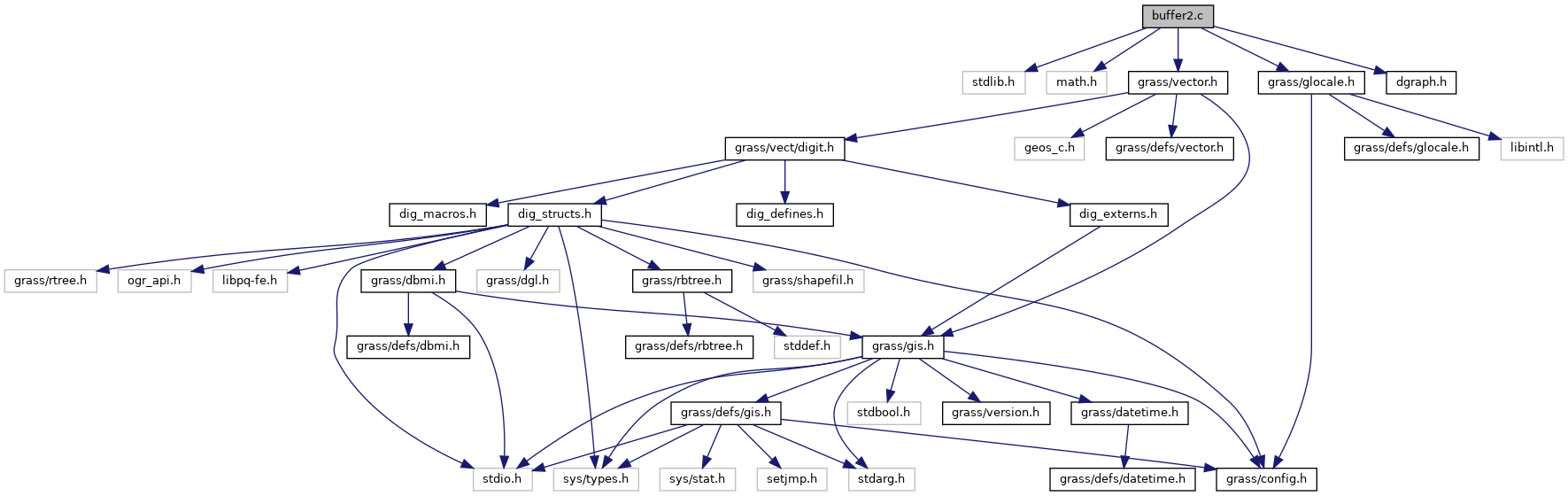GRASS GIS 7 Programmer's Manual  7.9.dev(2021)-e5379bbd7
buffer2.c File Reference

Vector library - nearest, adjust, parallel lines. More...

`#include <stdlib.h>`
`#include <math.h>`
`#include <grass/vector.h>`
`#include <grass/glocale.h>`
`#include "dgraph.h"`
Include dependency graph for buffer2.c:Go to the source code of this file.

## Macros

#define LENGTH(DX, DY)   (sqrt((DX*DX)+(DY*DY)))

#define MIN(X, Y)   ((X<Y)?X:Y)

#define MAX(X, Y)   ((X>Y)?X:Y)

#define PI   M_PI

#define RIGHT_SIDE   1

#define LEFT_SIDE   -1

#define LOOPED_LINE   1

#define NON_LOOPED_LINE   0

## Functions

void Vect_line_buffer2 (const struct line_pnts *Points, double da, double db, double dalpha, int round, int caps, double tol, struct line_pnts **oPoints, struct line_pnts ***iPoints, int *inner_count)
Creates buffer around line. More...

void Vect_area_buffer2 (const struct Map_info *Map, int area, double da, double db, double dalpha, int round, int caps, double tol, struct line_pnts **oPoints, struct line_pnts ***iPoints, int *inner_count)
Creates buffer around area. More...

void Vect_point_buffer2 (double px, double py, double da, double db, double dalpha, int round, double tol, struct line_pnts **oPoints)
Creates buffer around the point (px, py). More...

void Vect_line_parallel2 (struct line_pnts *InPoints, double da, double db, double dalpha, int side, int round, double tol, struct line_pnts *OutPoints)

## Detailed Description

Vector library - nearest, adjust, parallel lines.

Higher level functions for reading/writing/manipulating vectors.

(C) 2001-2009 by the GRASS Development Team

This program is free software under the GNU General Public License (>=v2). Read the file COPYING that comes with GRASS for details.

Definition in file buffer2.c.

## ◆ LEFT_SIDE

 #define LEFT_SIDE   -1

Definition at line 35 of file buffer2.c.

## ◆ LENGTH

 #define LENGTH ( DX, DY ) (sqrt((DX*DX)+(DY*DY)))

Definition at line 26 of file buffer2.c.

## ◆ LOOPED_LINE

 #define LOOPED_LINE   1

Definition at line 36 of file buffer2.c.

## ◆ MAX

 #define MAX ( X, Y ) ((X>Y)?X:Y)

Definition at line 31 of file buffer2.c.

## ◆ MIN

 #define MIN ( X, Y ) ((X

Definition at line 28 of file buffer2.c.

## ◆ NON_LOOPED_LINE

 #define NON_LOOPED_LINE   0

Definition at line 37 of file buffer2.c.

## ◆ PI

 #define PI   M_PI

Definition at line 33 of file buffer2.c.

Referenced by Vect_point_buffer2().

## ◆ RIGHT_SIDE

 #define RIGHT_SIDE   1

Definition at line 34 of file buffer2.c.

## ◆ Vect_area_buffer2()

 void Vect_area_buffer2 ( const struct Map_info * Map, int area, double da, double db, double dalpha, int round, int caps, double tol, struct line_pnts ** oPoints, struct line_pnts *** iPoints, int * inner_count )

Creates buffer around area.

Parameters
 Map vector map area area id da distance along major axis db distance along minor axis dalpha angle between 0x and major axis round make corners round caps add caps at line ends tol maximum distance between theoretical arc and output segments [out] oPoints output polygon outer border (ccw order) [out] inner_count number of holes [out] iPoints array of output polygon's holes (cw order)

Definition at line 1070 of file buffer2.c.

## ◆ Vect_line_buffer2()

 void Vect_line_buffer2 ( const struct line_pnts * Points, double da, double db, double dalpha, int round, int caps, double tol, struct line_pnts ** oPoints, struct line_pnts *** iPoints, int * inner_count )

Creates buffer around line.

Shape of buffer endings is managed by two parameters - round and cap. Setting round=1, cap=1 gives "classical" buffer, while round=0, cap=1 gives square end, but cap=0 – butt. See v.buffer manual or SVG stroke-linecap for examples.

To get "classical" buffer, set db equal to da, and dalpha to 0.

Parameters
 Points input line geometry da distance along major axis db distance along minor axis dalpha angle between 0x and major axis round make corners round (0 - square, not 0 - round) caps add caps at line ends (0 - butt, not 0 - caps) tol maximum distance between theoretical arc and output segments [out] oPoints output polygon outer border (ccw order) [out] iPoints array of output polygon's holes (cw order) [out] inner_count number of holes

Definition at line 1005 of file buffer2.c.

## ◆ Vect_line_parallel2()

 void Vect_line_parallel2 ( struct line_pnts * InPoints, double da, double db, double dalpha, int side, int round, double tol, struct line_pnts * OutPoints )

Definition at line 1188 of file buffer2.c.

References G_debug(), and line_pnts::n_points.

## ◆ Vect_point_buffer2()

 void Vect_point_buffer2 ( double px, double py, double da, double db, double dalpha, int round, double tol, struct line_pnts ** oPoints )

Creates buffer around the point (px, py).

Parameters
 px input point x-coordinate py input point y-coordinate da distance along major axis db distance along minor axis dalpha angle between 0x and major axis round make corners round tol maximum distance between theoretical arc and output segments [out] oPoints output polygon outer border (ccw order)

Definition at line 1135 of file buffer2.c.

References G_debug(), PI, and Vect_new_line_struct().